Next: Lagrangian and Euler Equations Up: Basic Equation of Fluid Previous: What is fluid?   Contents

# Equation of Motion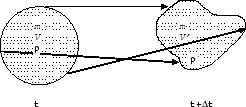Consider a fluid element with mass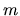as in A.1. From the Newton's second law of mechanics, the acceleration of an element is written as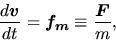(A.1)

where,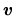,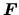, and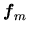represent the mass, the velocity of the element, and force working on the element and the force per mass working on the element, respectively.

In fluid dynamics, using the mass density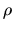and the force working on the unit volume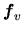equation (A.1) is rewritten as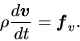(A.2)

Which kind of force works in a fluid? Gas pressure force does work in any fluids. Beside this, if there is the gravity,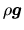should be included in. If the electric currents is running in the fluid and the magnetic fields exist, the Lorentz force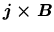should be added.

To write down the expression of the gas pressure force, consider a fluid element between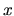and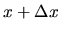as shown in Figure A.2. Pressure force exerting on the surface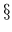atis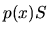, while that on the opposite side is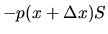. The net pressure force working on the volume of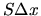is equal to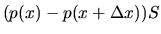, which is approximated as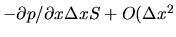).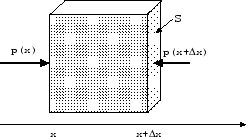Thus, the pressure force working on the unit volume is written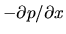. Equation (A.2) can be rewritten as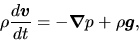(A.3)

when gravity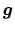is working.Next: Lagrangian and Euler Equations Up: Basic Equation of Fluid Previous: What is fluid?   Contents
Kohji Tomisaka 2007-07-08